# RC 积分器RC 积分器是串联的 RC 网络，其产生对应于积分的数学过程的输出信号。

### RC 积分器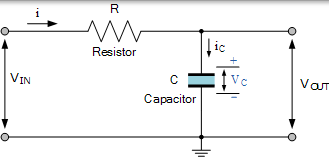$$i _ { C ( t ) } = C \frac { d V _ { C ( t ) } } { d t }$$

### RC 时间常数

$$\begin{array} { l } { \mathrm { RC } = \mathrm { R } \frac { \mathrm { Q } } { \mathrm { V } } = \mathrm { R } \frac { \mathrm { i } \times \mathrm { T } } { \mathrm { i } \times \mathrm { R } } = \mathrm { T } } \\ { \therefore \mathrm { T } = \mathrm { R } \mathrm { C } } \end{array}$$

## 电容器电压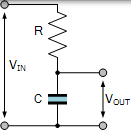Q =∫idt （任何时刻电容器上的电荷 Q）

$$\mathrm { i } ( \mathrm { t } ) = \frac { \mathrm { V } _ { \mathrm { IN } } } { \mathrm { R } } = \frac { \mathrm { V } _ { \mathrm { R } } } { \mathrm { R } } = \mathrm { C } \frac { \mathrm { d } V } { \mathrm { d } \mathrm { t } }$$

$$\mathrm { V } _ { \mathrm { OUT } } = \mathrm { V } _ { \mathrm { C } } = \frac { Q } { \mathrm { C } } = \frac { \int \mathrm { idt } } { \mathrm { C } } = \frac { 1 } { \mathrm { C } } \int \mathrm { i } ( \mathrm { t } ) \mathrm { d } \mathrm { t }$$

$$\mathrm { V } _ { \mathrm { OUT } } = \frac { 1 } { \mathrm { C } } \int \left( \frac { V _ { \mathrm { IN } } } { \mathrm { R } } \right) \mathrm { dt } = \frac { 1 } { \mathrm { R } \mathrm { C } } \int \mathrm { V } _ { \mathrm { IN } } \mathrm { d } \mathrm { t }$$

### RC 积分器公式

$$V _ { \mathrm { OUT } } = \frac { 1 } { \mathrm { R } C } \int _ { 0 } ^ { t } V _ { \mathrm { IN } ( \mathrm { t } ) } \mathrm { d } \mathrm { t }$$

## 单脉冲 RC 积分器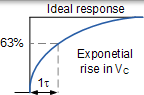$$V _ { C ( t ) } = \vee \left( 1 - e ^ { - \left( \frac { t } { R C } \right) } \right)$$

$$\mathrm { V } _ { \mathrm { C } ( \mathrm { t } ) } = \mathrm { V } \left( \mathrm { e } ^ { - \left( \frac { \mathrm { t } } { \mathrm { R } \mathrm { C } } \right) } \right)$$

0.5 39.4％ 60.6％
0.7 50％ 50％
1 63.2％ 36.7％
2 86.4％ 13.5％
3 95.0％ 4.9％
4 98.1％ 1.8％
5 99.3％ 0.67％

### RC 积分电路示例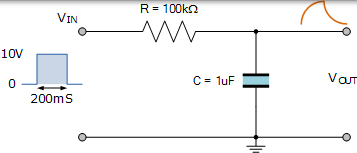### RC 积分器充电/放电曲线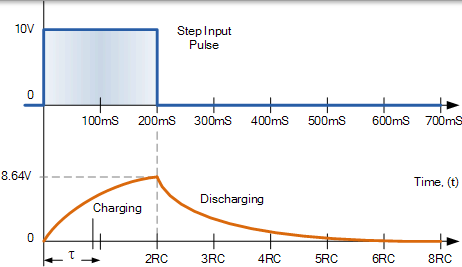### 固定 RC 积分器时间常数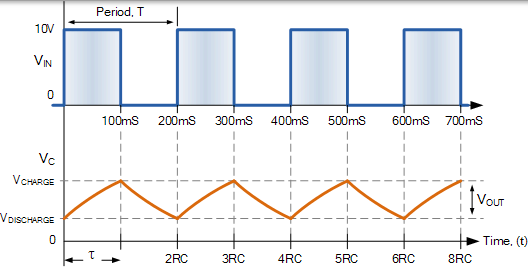## RC 积分器作为正弦波发生器

### 正弦波 RC 积分器## RC 积分器摘要

RC 积分器的时间常数总是与输入的周期 T 进行比较，因此长的 RC 时间常数将产生与输入信号相比具有低幅度的三角波形状，因为电容器具有较少的完全充电或放电时间。短时间常数允许电容器更多时间充电和放电，产生更典型的圆形形状。